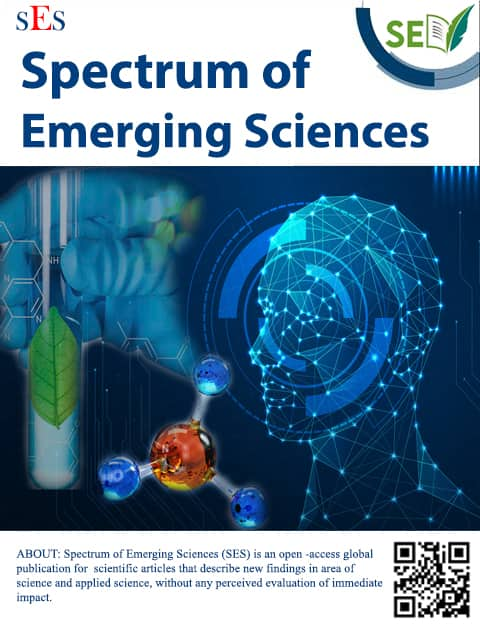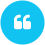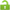ISSN:2583-2603

# Probabilistic Model for rGO-enriched nano-ceramics for electro-mechanical study

Author(s):

Email(s):

South Forsyth High School, Cumming, GA, 30041.Vania Munjar (2022). Probabilistic Model for rGO-enriched nano-ceramics for electro-mechanical study. Spectrum of Emerging Sciences, 2(2), pp. 6-9. 10.55878/SES2022-2-2-2View PDF

Please allow Pop-Up for this website to view PDF file.

I.     Introduction

Researchers studying the use of ceramic/graphene composites in diverse industrial applications include material scientists. Very few theoretical studies on the rGO in nano-ceramic matrix are published to date, despite substantial research on rGO nano-ceramics in diverse applications and in describing the rGO ceramics nano-structure. Because they are influenced by the proportion and orientation of rGO in the ceramic matrix, mechanical and electrical characteristics of rGO nano-ceramics are crucial.

A recent theoretical examination of the connection between the proportion of rGO in a ceramic matrix and the structure's electrical and mechanical characteristics is described in . This theoretical work increases the understanding of electrical conductivity and fracture toughness using sophisticated mathematical methodologies. It is possible to undertake a thorough theoretical investigation of the structure's structural entropy and electrical conductivity by expressing the rGO-included ceramic structure as a polyhedral complex. The paper also shows a theoretical method for figuring out the best rGO percentage to use in order to maximise structural and electrical attributes. The combinatorial structure of various orientations of rGO inclusion in ceramics matrix can lead to variable structural entropy and electrical conductivity values. The suggested method can produce electrical conductivity and structural entropy values that are ideal. The simulation study's findings support the theoretical framework of the rGO nano-ceramics analysis. Future studies can use this data to identify the proper proportion of rGO in the nano-ceramic matrix.

II. Preliminaries

Over the past 10 years, a large body of research has been conducted on reduced graphene oxide (rGO) ceramics, including multiple papers that have been published in a variety of publications. The development of applications for certain industries depends heavily on the utilization of rGO ceramics. The applicability and exploration of choosing the right rGO inclusion for certain applications are crucial for commercially viable products.

There are scientific and commercial uses for studying rGO/ceramic nano-composites. There have been very few theoretical investigations to better understand the behaviour of rGO nano-composites [1, 2], despite the fact that disconnected rGO's characteristics are well understood [2,]. The existing theoretical investigations in numerous research publications do not provide a strategy for logically building rGO composites with the necessary mix of mechanical and physical characteristics. The areas of materials science and design might greatly benefit from modern mathematical techniques. There are several techniques for modelling and characterising material design topologies in advanced discrete mathematics –. Recently, computational materials structure has made use of machine learning , neural networks , fractal theory , and biological pattern production . Because of the intriguing discrete topologies of connected 1D, 2D, and 3D substructures in bulk nanocrystalline composites, such as rGO plates, mathematical topology is very helpful in enhancing computer analysis. In material science and material design, contemporary mathematical methods are an invaluable tool. Bulk nano-crystalline composites with 2D inclusion of rGO plates may be described as complex discrete topologies of linked substructures.

A.     Polyhedron

The collection of solutions to a system of linear inequalities with non-negative variables that makes up a polyhedron may be written as,

……….

Hence, we can state that, a polyhedron P can be defined as a set such that

Numerous mathematical categories, including 0-dimensional faces (vertices), 1-dimensional faces (edges), 2-dimensional faces (planar polygons), 3-dimensional faces, and others, can be used to categorise the polyhedron's substructures. This is so that the polyhedron's topology of linked substructures may be articulated. We are now restricting the topological structure to a small convex 3-dimensional polyhedron in order to simplify the computational analysis.

III. Computational Model

A.     Polyhedral complex

A collection of polyhedra in a real vector space that fit together in a particular way are known as polyhedral complexes in mathematic . It is helpful to discretize complicated forms and see them as the union of basic building pieces that have been adhered together in a "clean way" in order to examine and handle them. Simple geometric shapes such as points, lines, segments of lines, triangles, tehrahedra, and more generally simplices, or even convex polytopes, should be used as the building blocks. Polyhedral complexes generalise simplicial complexes and appear in a number of polyhedral geometric situations, such as tropical geometry, splines, and hyperplane configurations. Regular quasi-convex discrete topological complexes are separated into polyhedral complexes [6, 12, 13], where 0 and 1 cells are represented by points or vertices, 2 and 3 cells by planar polygons, and so on. We are particularly interested in polyhedral 3-complexes with precisely three convex polyhedrons acting as 0-cells and three convex polyhedrons acting as 3-cells (Fig.1). Using the Voronoi tessellating approach, these complexes are created by surrounding each 1-cell with precisely three 2-cells and three 3-cells, and each 0-cell with precisely four 1-cells, six 2-cells, and four 3-cells . This kind of mathematical description is widely utilised in molecular dynamics simulations – because it precisely mimics the microstructures of actual materials.

Fig 1: Polyhedral complex and its elements 

B.     Structural entropy

The 2-cells of the 3-complex being occupied or not by rGO plates serves as a representation of the rGO/ceramic nanocomposite microstructure. It is reasonable to compare rGO plates to 2-cells since their breadth is just a few atomic layers, which is insignificant when compared to the size of the 3-cells. Calculating the rGO-occupied 2-cells requires knowing the proportion (p) of feasible 2-cells in the structure. pN2 is the combinatorial realisation of rGO inclusion in an N-sized vector, where N2 is the total number of 2-cell configurations that are feasible. rGO 2-cells' pN2 number may be the consequence of several geometric realisations. Based on the quantity of rGO inclusions, the 1-cell may be divided into four different categories, just as the 2-cell.For the sake of simplicity, consider the four types of 1-cells as Jω for ω = 0,1,2,3.

Fig 2: Triple junction of rGO plate

As per , the structural entropy can be described as,

This definition is closely related to the Shannon informational entropy  and, on the other hand, to the alloy configurational entropy .

C.     Conductivity

Electrical conductivity is the main physical characteristic of interest in nanostructured ceramics. The conductivity and fraction p are inversely related. The 2-cell random distribution provided in rGO is a rather straightforward and well-developed issue. The proportion of peculiar 2-cells defines the percolation threshold in this example to be p = 0.08. Above the threshold, the graph's conductivity exhibits a quasilinear dependency on p as,

Here   for considered 3-complex .  is the conductivity for p = 1 and H represents the Heaviside step function. The equation shows a linear growth of conductivity at large enough p

IV. Simulation results

For the polyhedral complex, we have simulated the structure using 500X500 odes in which 2-cell nodes are of  fraction and  p fraction of f represents the number of rGO inclusions in the matrix. We can iterate the the structure with different random configuration and can obtain the conductivity value for various p and f values at each iteration of the process. The theoretical curve of  rGO portion vs Structural Entropy is as follows (fig 3):

Figure 3: rGO portion vs Structural Entropy

Whereas rGO portion vs Conductivity is linear except of those values where the Heaviside function is considered zero (fig 4).

Figure 4: RGO portion vs Conductivity

Using the given equation,  the structural entropy  and conductivity  can be found as fig 5 (a). The simulation runs for various random configurations with different p and f values. The analysis requires the maximum conductivity in each fixed p and f. The structural entropy vs conductivity values for a Gaussian distribution are shown in fig 5.(b):

(a)

(b)

V. Conclusion

Recent research  demonstrates the usefulness of cutting-edge mathematical theory in comprehending the nano-structure of rGO, including ceramics. In this study, the structural entropy and conductivity of the rGO nano-ceramics were examined for various random configurations using combinatorial analysis on the random matrix structure. A crucial factor in determining the optimal value of structural entropy and conductivity is the proportion of rGO incorporation. Here, a simulation research is conducted to comprehend how structural entropy and conductivity behave in order to find the optimal conductivity solution.

## Recomonded Articles:

### Image Fusion using Wavelet Transform: Systematic Literature Review

Author(s): Sushant Bindra; Mehak Piplani

DOI:         Access:Open Access Read More

### Effect of Plasticizer Concentration on the Physicochemical Properties of Starch-based Bioplastic Prepared from Potato Peels

Author(s): Binod Shrestha, Sambridhi Shah, Khagendra Chapain, Rajendra Joshi, Rajesh Pandit

DOI: 10.55878/SES2022-2-1-7         Access:Open Access Read More

### Thermal analysis of Exhaust manifold system using computational fluid dynamics

Author(s): Anand Pathak; Saurabh Deshmukh

DOI:         Access:Open Access Read More

### PDE based Analysis for Propagation of Disturbance by Users Mobility in Mobile Network System

Author(s): Shathya Pranav Sujithra Rajesh Kannan

DOI: 10.55878/SES2022-2-2-1         Access:Open Access Read More

### Probabilistic Model for rGO-enriched nano-ceramics for electro-mechanical study

Author(s): Vania Munjar

DOI: 10.55878/SES2022-2-2-2         Access:Open Access Read More